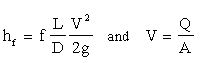Darcy-Weisbach Pipe Friction Loss Calculator

Compute Major Losses (Friction Loss) in Pipes using Darcy-Weisbach Equation

 Solve for: Circular Duct: Enter D and V Circular Duct: Enter D and Q Non-circular Duct: Enter A, P, and V Non-circular Duct: Enter A, P, and Q Moody Friction Factor, f: Select units: Duct Length, L (ft): Use feet and seconds units Use meters and seconds units Duct Diameter, D (ft): © 2014 LMNO Engineering, Duct Area, A (ft2): Research, and Software, Ltd. Duct Perimeter, P (ft): http://www.LMNOeng.com Velocity, V (ft/s): Discharge, Q (ft3/s): Major Loss, hf (ft):

Units in Darcy-Weisbach calculator: ft=foot, m=meter, s=second.

Darcy-Weisbach Friction Loss Equation:D is called the "duct diameter" to keep the terminology general to include circular pipes and non-circular pipes, also known as ducts. For rectangular pipes (ducts), D=4A/P is known as the hydraulic diameter. More information about hydraulic diameter can be found on our non-circular to circular pipe conversion page.

g = acceleration due to gravity = 32.174 ft/s2 = 9.806 m/s2.

Major loss (hf) is the energy (or head) loss (expressed in length units - think of it as energy per unit weight of fluid) due to friction between the moving fluid and the duct. It is also known as friction loss. The Darcy-Weisbach method is generally considered more accurate than the Hazen-Williams method. Additionally, the Darcy-Weisbach method is valid for any liquid or gas; Hazen-Williams is only valid for water at ordinary temperatures (40 to 75 oF). The Hazen-Williams method is very popular, especially among civil engineers, since its friction coefficient (C) is not a function of velocity or duct diameter. Hazen-Williams is simpler than Darcy-Weisbach for calculations where you are solving for flowrate, velocity, or diameter. More Discussion and References.

The Darcy-Weisbach calculation does not check for unreasonable inputs such as negative values. All values should be entered as positive.

© 1998-2014 LMNO Engineering, Research, and Software, Ltd.  All rights reserved.

LMNO Engineering, Research, and Software, Ltd.
7860 Angel Ridge Rd.   Athens, Ohio 45701  USA   Phone: (740) 592-1890
LMNO@LMNOeng.com    http://www.LMNOeng.com

To:
LMNO Engineering home page (more calculations)

Pipe Design (Darcy-Weisbach friction losses)

Moody friction factor

Unit Conversions

Other information:

Register

Discussion and References